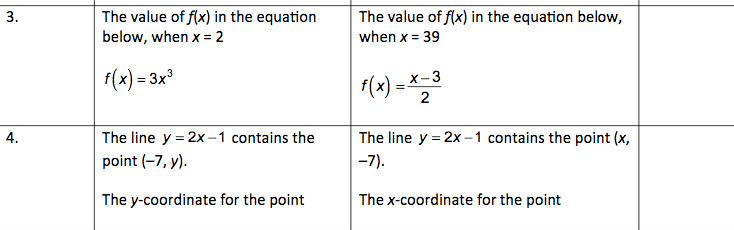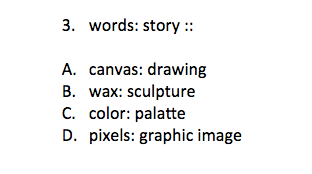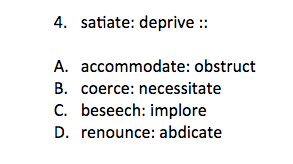Start Now with 100 Free Questions

# SCAT 6th – 8th Grade Practice Test

## Test Structure

The SCAT is divided into two sections – Verbal and Quantitative. Each section consists of 55 questions, out of which 5 are experimental and not scored. These two sections test the reasoning skills of the student rather than specific knowledge in certain subjects.

• Verbal Section: This section measures understanding of the meaning of words and verbal reasoning ability. The format is a verbal analogy which requires selecting the answer choice that best completes the analogy.
• Quantitative Section: This section measures mathematical reasoning ability. It uses mathematical comparisons where a relationship between two quantities is given, and the student needs to determine a similar relationship from four given options.

Each section of the test lasts approximately 22 minutes. There is a 10-minute break in between the two sections. The entire testing process, including administrative tasks, takes about 1 hour.

### 1.) Quantitative Section

Directions: Each of the following questions has two parts. Look at the part in Column A. Then look at the part in column B. Decide if one part is greater then the other or if the parts are equal. Then, choose one of the four answers below and write it into the 4th column:

A- If the part in Column A is greater

B- If the part in Column b is greater

C- If the two parts are equal

D-  Not enough information to decide### 2.) Verbal Section

Directions: Look at the two words on top. They belong together in a certain way. Now look at the answer choices below. Choose the best pair of words in the answer choices that go together the same way the two words on top go together.Quantitative Section

1.) A > 18

2.) -15 < -3

Verbal Section

1.) D- Individual parts of whole

2.) A-Antonym

3 Responses

Thank you

Hello,

If I become a member, how many sets of SCAT test for 6-8th graders (or up? my child is a 9th grader) can I have access to? Do you also provide answer keys?

Thanks.Hi Xubei –

Thank you for reaching out to us! With a membership, you currently have access to 2 advanced level math practice tests and 3 advanced level verbal practice tests. These practice tests are printable and answer keys are provided.

In addition to our printable practice questions, we also provide interactive practice questions for the SCAT.

All the best,

Marcus

See if TestingMom.com supports your child’s test by your school district. If you don't see your child's school district listed, check with us! We have practice for other tests as well.